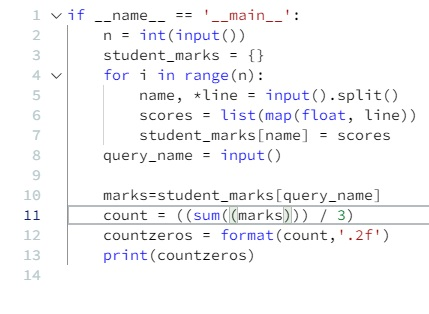# Hackerrank – Python – Finding the percentage – Solution

Publish Date - February 23rd, 2023

|

Another great solution that is not only mostly done for you, but really pokes at your brain to solve the right solution. Knowing data types is important for any OOP like Java, JavaScript, C++, PHP and many others. Knowing how to cast and manipulate data types are also important for data science and machine learning since Python is a regularly used language for those disciplines. Anyways, I hope this solution helps you in your Python journey!

## The problem

The provided code stub will read in a dictionary containing key/value pairs of name:[marks] for a list of students. Print the average of the marks array for the student name provided, showing 2 places after the decimal.

Example
marks key: value pairs are

‘alpha’: [20, 30, 40]

‘beta’: [30, 50, 70]

query_name = ‘beta’

The query_name is ‘beta’. beta’s average score is (30 + 50 + 70) / 3 = 50.0

Input Format

The first line contains the integer , the number of students’ records. The next  lines contain the names and marks obtained by a student, each value separated by a space. The final line contains query_name, the name of a student to query.

Output Format

Print one line: The average of the marks obtained by the particular student correct to 2 decimal places.

Sample Input 0

```3
Krishna 67 68 69
Arjun 70 98 63
Malika 52 56 60
Malika
```

Sample Output 0

```56.00
```

Explanation 0

Marks for Malika are  [52, 56, 60] whose average is (52 + 56 + 60) / 3 = 56

Sample Input 1

```2
Harsh 25 26.5 28
Anurag 26 28 30
Harsh
```

Sample Output 1

`26.50`

## The solution

``````if __name__ == '__main__':
n = int(input())
student_marks = {}
for i in range(n):
name, *line = input().split()
scores = list(map(float, line))
student_marks[name] = scores
query_name = input()

# my code below
marks=student_marks[query_name]
count = ((sum((marks))) / 3)
countzeros = format(count,'.2f')
print(countzeros)
``````

The first 9 lines of code or so are generated by HackerRank so you don’t need to worry about that. However having a strong understanding of the problem will really illuminate the what you need to code.

This problem is essentially saying:

Based off a set of inputs:

• a number
• some names with a list of integers
• a name at the end

I want the average of the name highlighted at the end based off the number of inputs provided.

Knowing that, you already know a few things:

• need to do some math functions (SUM or AVG)
• need to create a variable based mapping the query_name input to a set a student marks (important)
• you may need to format the number because the final result has two decimal point zeros (float automatically goes to one).

Based off the logic above you have the following:

``````    marks=student_marks[query_name] <= student_marks mapped to query_name = marks
count = ((sum((marks))) / 3) <= add-up all of the marks (don't forget your BEDMAS) and divide the value by 3.
countzeros = format(count,'.2f') <= format the average to add two sets of zeroes.
print(countzeros) <= output``````

Your output for both queries should be:

You should get sample outputs for the unit tests:

``56.00``
``26.50``

Be careful of those second set of zeroes after the decimal place. You will 100% have to format that in.

I hope this helps your journey to learn Python!